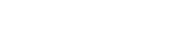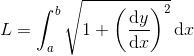# Category: Calculus## Arc length formula

Today we are going to find the formula that allows us to calculate the arc length given a function f(x). This is quite intuitive, so you should have no problem!… Read more Arc length formula## Integral of sin(x)/x from 0 to infinity | A satisfying solution

Today we have a tough integral: not only is this a special integral (the sine integral Si(x)), but it also goes from 0 to infinity! Because of the first characteristic,… Read more Integral of sin(x)/x from 0 to infinity | A satisfying solution## Integral of e^(ax)*sin(x)

In this post we are going to integrate this seemingly hard integral, which in my opinion is actually very interesting! When we have a product of a trigonometric and a… Read more Integral of e^(ax)*sin(x)## Integral of sec²(x)/exp(tan(x))

Although it may look a bit intimidating at first, I can guarantee you this is actually a very easy integral! But what integration technique do you think will be useful?… Read more Integral of sec²(x)/exp(tan(x))## Half integral of x

In my previous post I showed you how to take the half derivative of x. Here, I am going to explain how to find the half integral of x. We… Read more Half integral of x## Half derivative of x

The derivative of x is easy, it’s simply 1; the second derivative of x is the derivative of 1, which is 0; but what on earth is the half derivative… Read more Half derivative of x## Cauchy principal value | Definite integral of 1/x

We know that the integral of 1/x is the natural logarithm ln(x). Very straightforward, yes, but it’s not as simple when it comes to evaluating the definite integral. As you… Read more Cauchy principal value | Definite integral of 1/x## Integral of arcsin(x)

In this post I am going to show you how to integrate the inverse function of sin(x), arcsin(x), step-by-step. But first, let’s clear a few things. What is arcsin(x)? Arcsine… Read more Integral of arcsin(x)

{{#pages}} {{/pages}}
%%footer%%